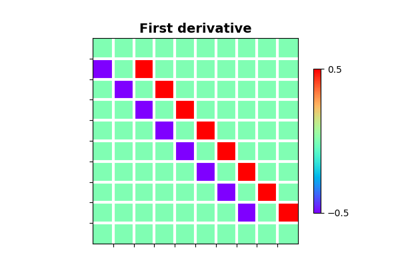# pylops.FirstDirectionalDerivative¶

pylops.FirstDirectionalDerivative(dims, v, sampling=1, edge=False, dtype='float64', kind='centered')[source]

First Directional derivative.

Apply a directional derivative operator to a multi-dimensional array along either a single common direction or different directions for each point of the array.

Note

At least 2 dimensions are required, consider using pylops.FirstDerivative for 1d arrays.

Parameters: dims : tuple Number of samples for each dimension. v : np.ndarray, optional Single direction (array of size $$n_\text{dims}$$) or group of directions (array of size $$[n_\text{dims} \times n_{d_0} \times ... \times n_{d_{n_\text{dims}}}]$$) sampling : tuple, optional Sampling steps for each direction. edge : bool, optional Use reduced order derivative at edges (True) or ignore them (False). dtype : str, optional Type of elements in input array. kind : str, optional Derivative kind (forward, centered, or backward). ddop : pylops.LinearOperator First directional derivative linear operator

Notes

The FirstDirectionalDerivative applies a first-order derivative to a multi-dimensional array along the direction defined by the unitary vector $$\mathbf{v}$$:

$df_\mathbf{v} = \nabla f \mathbf{v}$

or along the directions defined by the unitary vectors $$\mathbf{v}(x, y)$$:

$df_\mathbf{v}(x,y) = \nabla f(x,y) \mathbf{v}(x,y)$

where we have here considered the 2-dimensional case.

This operator can be easily implemented as the concatenation of the pylops.Gradient operator and the pylops.Diagonal operator with $$\mathbf{v}$$ along the main diagonal.

## Examples using pylops.FirstDirectionalDerivative¶Derivatives